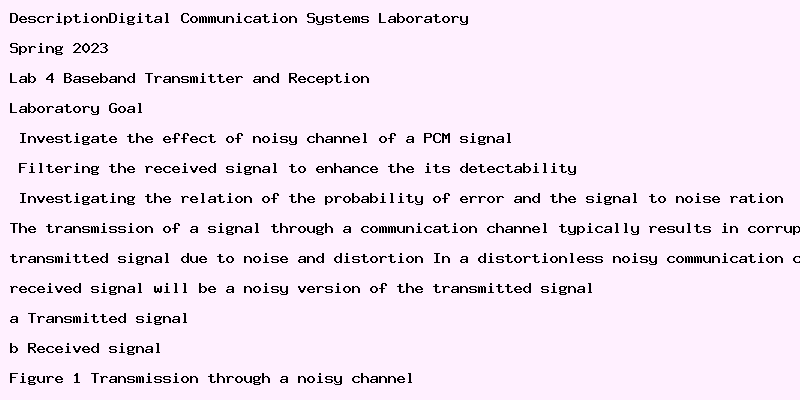# Temple University Matlab of Baseband Transmitter and Reception Lab Report

DescriptionDigital Communication Systems Laboratory
Spring 2023
Lab 4: Baseband Transmitter and Reception
Laboratory Goal
• Investigate the effect of noisy channel of a PCM signal.
• Filtering the received signal to enhance the it’s detectability.
• Investigating the relation of the probability of error and the signal to noise ration.
The transmission of a signal through a communication channel typically results in corruption of the
transmitted signal due to noise and distortion. In a distortionless noisy communication channel, the
received signal will be a noisy version of the transmitted signal.
(a) Transmitted signal
Figure 1: Transmission through a noisy channel
1
Direct sampling the noisy signal does not take advantages of the total energy transmitted over the time
interval of T. So, we need to do some kind of filtering.
For a signal g(t),the impulse response of the optimal filter is
hopt (t) = kg(T − t),
which is call a matched filter.
(a) Rectangular pulse
(b) Matched filter output
Figure 3: Matched filtering of a rectangular pulse
Figure 4: Receiver for baseband transmission of binary wave using NRZ signaling
2
a. Amplitude ((A): (TUID(9) + 2) Volt.
b. Frequency (f ): (TUID(8) + 3) KHz
1. Change the SNR value for 20 dB to −20 dB with an interval of 2 dB to calculate the error rate.
plot the error rate against SNR.
2. Consider a transmitted signal encoded with Unipolar non return to zero scheme. Plot the transmitted, received and filtered signal for 0 dB SNR. what is the error rate?
1. Repeat Task 2 for Manchester coding.
3
clear
close
clc
%%
A = 1; % Amplitude
rb = 1000; % bit rate
Tb = 1/rb; % bit interval
fs = 100*rb; % Sampling rate
Ts = 1/fs; % Sampling Interval
M = 2; % Numbe of symbols
N = 30; % Number of bits to be transmitted
Ns = floor(Tb/Ts); % Number of samples per bit
pulse = ones(1,Ns); % Reference pulse
figure(1)
stem(t1,pnrz);
xlabel(‘Time’)
ylabel(‘Amplitude’)
figure(2)
plot(t2,signal_prnz)
xlabel(‘Time’)
ylabel(‘Amplitude’)
%% Noisy Channel
snr_db = 0; % signal to noise power in db
received = awgn(signal_prnz,snr_db); % Passing the signal through a noisy channel
figure(3)
xlabel(‘Time’)
ylabel(‘Amplitude’)
%% Matched filtering
filtered_signal = zeros(1,N);
% Performing matched filtering
for i = 1:length(signal_prnz)/Ns
idx = (i-1)*Ns+1:(i-1)*Ns+Ns;
filtered_signal(i) = filtered(Ns);
end
filtered_signal = filtered_signal/(A^2*Ns);
%% Detection
detected = filtered_signal;
detected(filtered_signal>=0)=1;
detected(filtered_signal
attachment#### Why Choose Us

• 100% non-plagiarized Papers
• Affordable Prices
• Any Paper, Urgency, and Subject
• Will complete your papers in 6 hours
• On-time Delivery
• Money-back and Privacy guarantees
• Unlimited Amendments upon request
• Satisfaction guarantee

#### How it Works

• Click on the “Place Your Order” tab at the top menu or “Order Now” icon at the bottom and a new page will appear with an order form to be filled.
• Fill in your paper’s requirements in the "PAPER DETAILS" section.